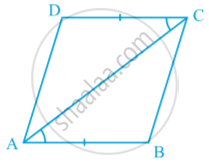# Another Condition for a Quadrilateral to Be a Parallelogram

#### theorem

Theorem: A quadrilateral is a parallelogram if a pair of opposite sides is equal and parallel.In above fig. which is AB = CD and AB || CD .  Let us draw a diagonal AC. You can show that ∆ ABC ≅ ∆ CDA by SAS congruence rule. So ,  BC || AD .

If you would like to contribute notes or other learning material, please submit them using the button below.

### Shaalaa.com

Theorem : A quadrilateral is a parallelogram if a pair of opposite sides is equal and parallel. [00:12:07]
S